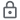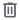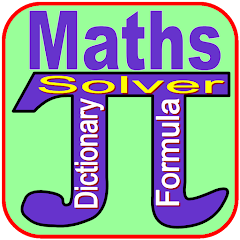# Maths Dictionary And Formula

10K+Everyone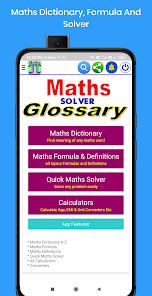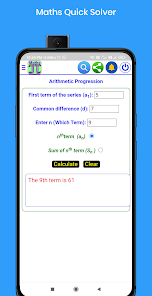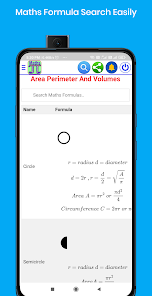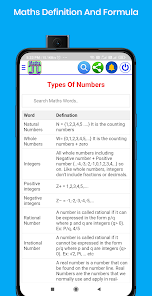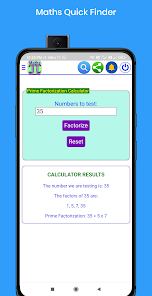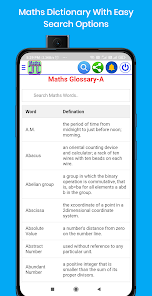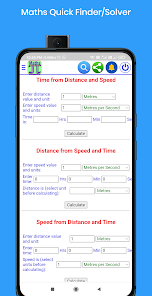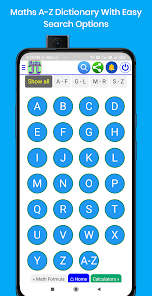Maths Dictionary And Formula is a complete app for All students and teachers.

Maths Dictionary, Formula And Quick Solver App Features:
* Maths Dictionary with A-Z Easy Search Options.
* Maths All Class Topic Wise Formula with Easy Search Options.
* Maths Quick Solver/ Finder to solve any questions/problems.
* Maths Definitions.
* All Type Of Calculators Like: Basic, Scientific, EMI and Age Calculators Etc.
* Unit Converters Like: Length, Area, Speed, Weight, Volume and Temperature in Different Units.
* For All Easy and beautiful User Interference.

Maths Formula And Definitions included in the App:
* Number System
* Fractions
* Algebra
* Sets
* Progressions
* Polynomials
* Pair Of Linear Equations
* Coordinate Geometry
* Trigonometry
* Application Of Trigonometry
* Statistics
* Arithmetics

Maths Quick Solver/Finder Included in the APP:
* Divisibility Checker
* Factors Finder
* Prime Number Checker
* Prime Factorisation Finder
* Roman Numeral Converter
* Squar And Square Root Finder
* Cube And Cube Root Finder
* Find Nth Root of any number
* LCM and GCD/HCF Solver
* Percentage Calculator
* Percent Increaser
* Simple Interest Calculator
* Compund Interest Calculator
* Find Profit And Loss
* Find Proportion of four numbers
* Speed, Time And Distance Finder
* Group Work of Mens Questions Solver
* Area, Perimeter, Circumference and Volume calculator
* Solve Pythagoras theorem
* Distance from point A to point B
* Mid Point Finder
* Centroid of triangle of three points
* Find Area Of triangle of three points
* Slope of line problem Solve
* Find point slope form of equation
* Arithmetic Progression Finder
* Geometric Progression Solver

Note: Maths Dictionary, Formula And Solver App developed by Baigacademy.Maths Dictionary, Maths Formula, Quick Solver, Calculators & Unit Converters are prepared by Baigacademy. We tried our total efforts to present app without errors. but may be some errors occurred in the app and material. if found any errors and for your suggestion please mail us
Updated on
Dec 6, 2022

## Data safety

Safety starts with understanding how developers collect and share your data. Data privacy and security practices may vary based on your use, region, and age. The developer provided this information and may update it over time.This app may share these data types with third parties
App info and performance and Device or other IDsNo data collected